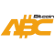Bitcoin ABC  0.22.12 P2P Digital Currency
compressor.cpp File Reference
`#include <compressor.h>`
`#include <pubkey.h>`
`#include <script/standard.h>`
Include dependency graph for compressor.cpp:

Go to the source code of this file.

## Functions

static bool IsToKeyID (const CScript &script, CKeyID &hash)

static bool IsToScriptID (const CScript &script, CScriptID &hash)

static bool IsToPubKey (const CScript &script, CPubKey &pubkey)

bool CompressScript (const CScript &script, std::vector< uint8_t > &out)

unsigned int GetSpecialScriptSize (unsigned int nSize)

bool DecompressScript (CScript &script, unsigned int nSize, const std::vector< uint8_t > &in)

uint64_t CompressAmount (Amount amt)

Amount DecompressAmount (uint64_t x)

## ◆ CompressAmount()

 uint64_t CompressAmount ( Amount amt )

Definition at line 150 of file compressor.cpp.

Here is the caller graph for this function:

## ◆ CompressScript()

 bool CompressScript ( const CScript & script, std::vector< uint8_t > & out )

Definition at line 53 of file compressor.cpp.

Here is the call graph for this function:
Here is the caller graph for this function:

## ◆ DecompressAmount()

 Amount DecompressAmount ( uint64_t x )

Definition at line 170 of file compressor.cpp.

Here is the call graph for this function:
Here is the caller graph for this function:

## ◆ DecompressScript()

 bool DecompressScript ( CScript & script, unsigned int nSize, const std::vector< uint8_t > & in )

Definition at line 93 of file compressor.cpp.

Here is the call graph for this function:
Here is the caller graph for this function:

## ◆ GetSpecialScriptSize()

 unsigned int GetSpecialScriptSize ( unsigned int nSize )

Definition at line 83 of file compressor.cpp.

Here is the caller graph for this function:

## ◆ IsToKeyID()

 static bool IsToKeyID ( const CScript & script, CKeyID & hash )
static

Definition at line 19 of file compressor.cpp.

Here is the call graph for this function:
Here is the caller graph for this function:

## ◆ IsToPubKey()

 static bool IsToPubKey ( const CScript & script, CPubKey & pubkey )
static

Definition at line 38 of file compressor.cpp.

Here is the call graph for this function:
Here is the caller graph for this function:

## ◆ IsToScriptID()

 static bool IsToScriptID ( const CScript & script, CScriptID & hash )
static

Definition at line 29 of file compressor.cpp.

Here is the call graph for this function:
Here is the caller graph for this function: